Question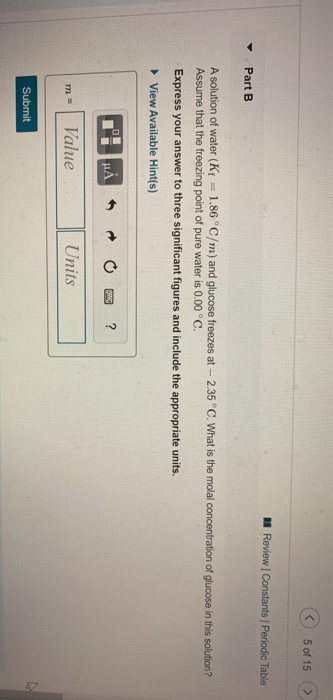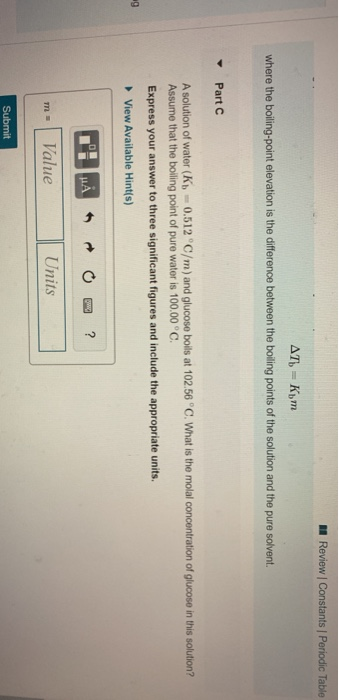part b and c please

part B

dT = Kf x m, where d = delta (change), Kf is the freezing point depression constant, and m = molality.

[0-(-2.35)]oC = 1.86 oC/m x m

m = 2.35 oC / 1.86 oC/m = 1.263 molal

part C

dT = Kb x m, where d = delta (change), Kb is the boiling point elevation constant, and m = molality.

[102.56-100.00] oC = 0.512 oC/m x m

m = 2.56 oC / 0.512 C/m = 5.00 molal

#### Earn Coins

Coins can be redeemed for fabulous gifts.

Similar Homework Help Questions
• ### Molality, Freezing Point, and Boiling Point 29 of 44 - Part 3 Review Constants Periodic Table...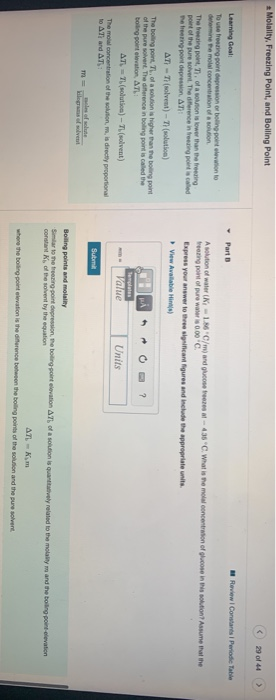Molality, Freezing Point, and Boiling Point 29 of 44 - Part 3 Review Constants Periodic Table Learning Goal Toute ring point depression or boling point elevation to din Pemola concentration of a solution The bring point, T. of a sortion is lower than the freezing point of the pure solvent. The difference in freezing point is called The treening point depression. AT AT - (solvent) - Tolution) The big pont, Th. of a solution is higher than the boting point...

• ### Part B A solution of water (kr = 1.86°C/m) and glucose freezes at - 415°C What...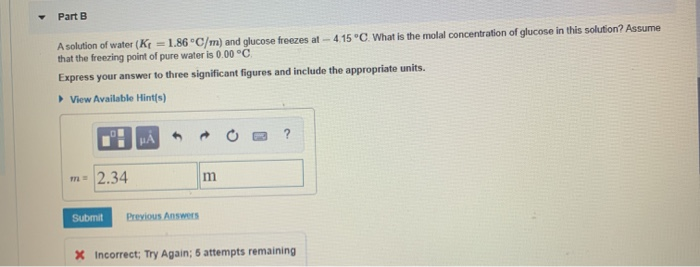Part B A solution of water (kr = 1.86°C/m) and glucose freezes at - 415°C What is the molal concentration of glucose in this solution? Assume that the freezing point of pure water is 0.00 °C Express your answer to three significant figures and include the appropriate units. View Available Hint(s) m= 2.34 m Submit Previous Answers X Incorrect: Try Again: 5 attempts remaining Part A solution of water (K = 0.512 °C/m) and glucose boils at 101.56 °C. What...

• ### A Review Constants Periodic Table The changes in boiling point (AT) or freezing point (AT) in...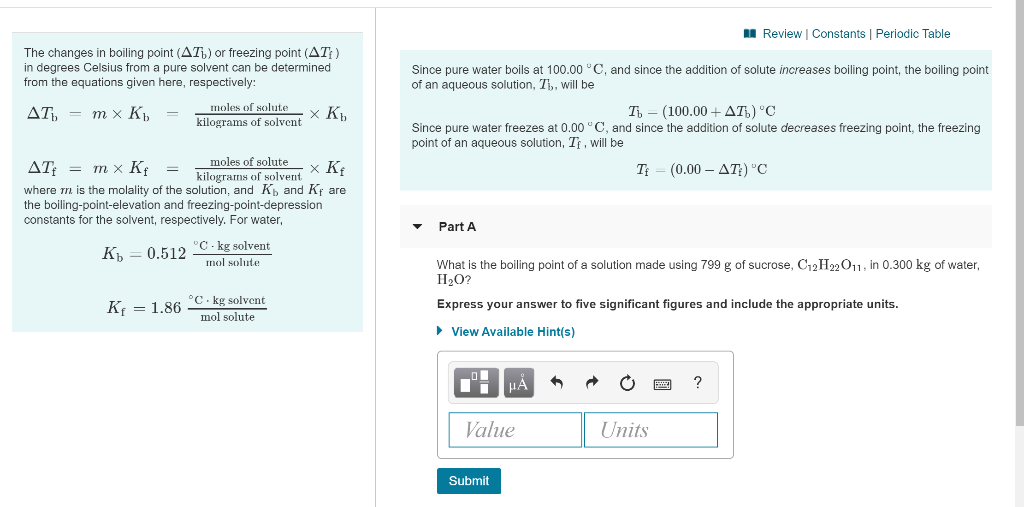A Review Constants Periodic Table The changes in boiling point (AT) or freezing point (AT) in degrees Celsius from a pure solvent can be determined from the equations given here, respectively: AT) = m x K = moles of solute XK K. kilograms of solvent Since pure water boils at 100.00 °C, and since the addition of solute increases boiling point, the boiling point of an aqueous solution, Th, will be T - (100.00+AT) 'C Since pure water freezes at...

• ### Review Constants Periodic Table The changes in boiling point (AT) or freezing point (AT) in degrees...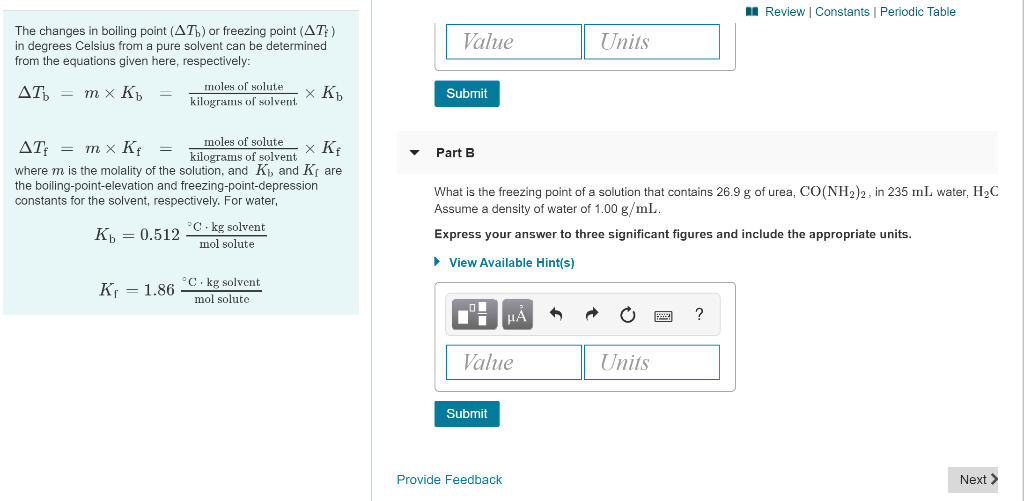Review Constants Periodic Table The changes in boiling point (AT) or freezing point (AT) in degrees Celsius from a pure solvent can be determined from the equations given here, respectively: Value Units moles of solute AT = mx Kb = 7 Submit kilograms of solvent XRb moles of solutex Kf Part B AT: = mx Kf = kilograms of solvent where m is the molality of the solution, and K and K the boiling-point-elevation and freezing-point-depression constants for the solvent,...

• ### Review Constants 1 Periodic Table The concentration of OH in a sample of seawater is 1.2x10-6...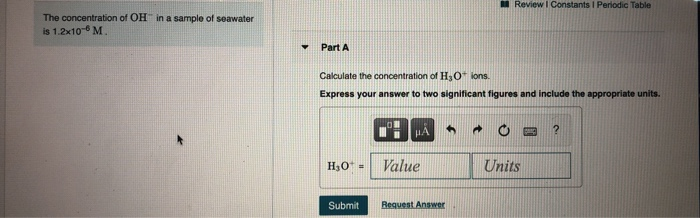Review Constants 1 Periodic Table The concentration of OH in a sample of seawater is 1.2x10-6 M Y Part A Calculate the concentration of H3O+ ions. Express your answer to two significant figures and include the appropriate units. HA ? H0+ - Value Units Submit Request Answer Review Constants ! Periodic Table Water Ionizes by the equation Part A H2O(1) H+ (aq) + OH(aq) The extent of the reaction is small in pure water and dilute aqueous solutions. This reaction...

• ### Answer Part A-C please, thanks. II Review | Constants | Periodic Table A 0.32 mol amount...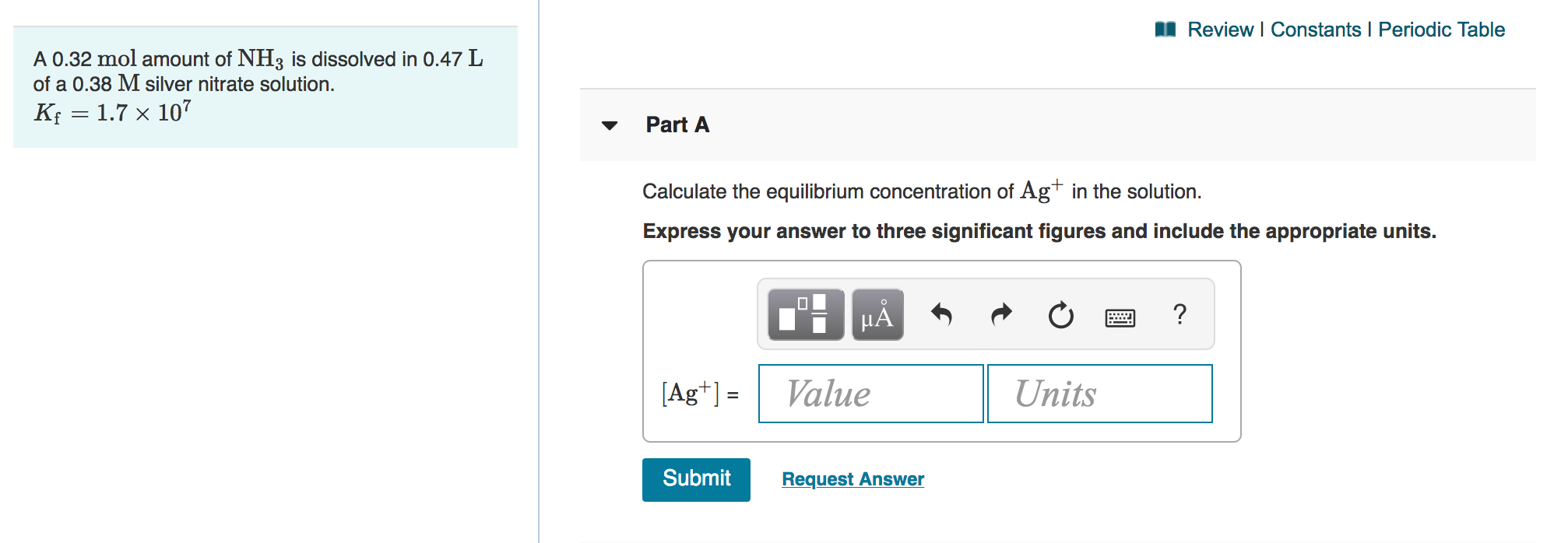Answer Part A-C please, thanks. II Review | Constants | Periodic Table A 0.32 mol amount of NH3 is dissolved in 0.47 L of a 0.38 M silver nitrate solution. Kf = 1.7 x 107 Part A Calculate the equilibrium concentration of Ag+ in the solution. Express your answer to three significant figures and include the appropriate ? OCH HA Value O Units [Ag*)= Submit Request Answer Part B Calculate the equilibrium concentration of NH3 in the solution. Express your...

• ### < on 10 of 28 A Review Constants Periodic Table Part A What is the boiling...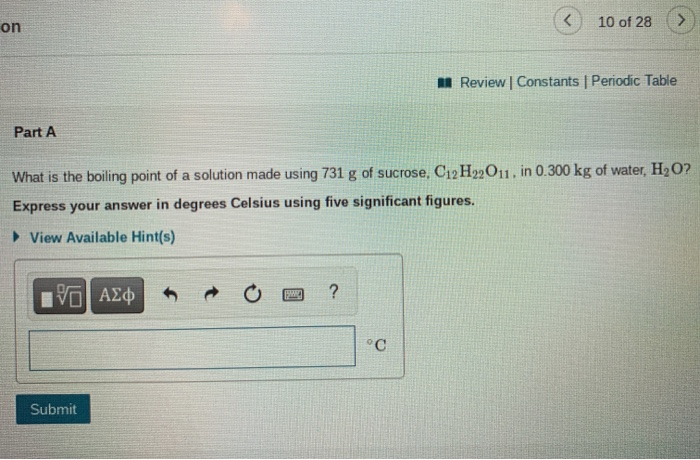< on 10 of 28 A Review Constants Periodic Table Part A What is the boiling point of a solution made using 731 g of sucrose, C12H22011. in 0.300 kg of water, H2O? Express your answer in degrees Celsius using five significant figures. View Available Hint(s) VO AO ? °C Submit Part B What is the freezing point of a solution that contains 26.4 g of urea, CO(NH2)2. in 225 mL water, H2O? Assume a density of water of 1.00...

• ### Review I Constants 1 Periodic Table Part B What is the final volume V2 in milliliters...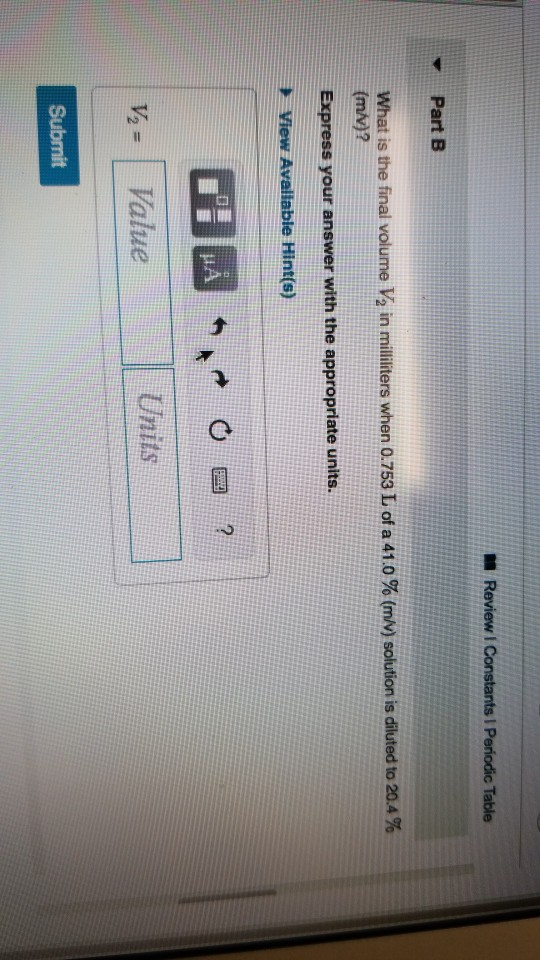Review I Constants 1 Periodic Table Part B What is the final volume V2 in milliliters when 0.753 L of a 41.0 % (m/v) solution is diluted to 20.4 % (m/v)? Express your answer with the appropriate units. View Available Hint(s) DA *MAN OR? Value Units V = Submit Review | Constants 1 Periodic Table Part C A 841 ml NaCl solution is diluted to a volume of 1.46 L and a concentration of 8.00 M. What was the initial...

• ### Exercise 7.118 11 of 23 > Review Constants Periodic Table Part A Apure gold ning and...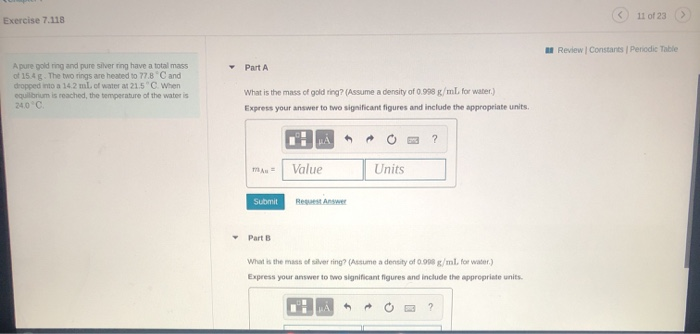Exercise 7.118 11 of 23 > Review Constants Periodic Table Part A Apure gold ning and pure silver ring have a total mass of 15.4g. The two rings are heated to 778 and dropped into a 14.2 ml.of water a 21.5 When equilibrium is reached, the temperature of the water is 2000 What is the mass of gold ring? (Assume a density of 0.998 g/ml for water.) Express your answer to two significant figures and include the appropriate units. mu...

• ### 13 of 25 Review | Constants Periodic Ta Part A 9.00x10- mol of HBr are dissolved...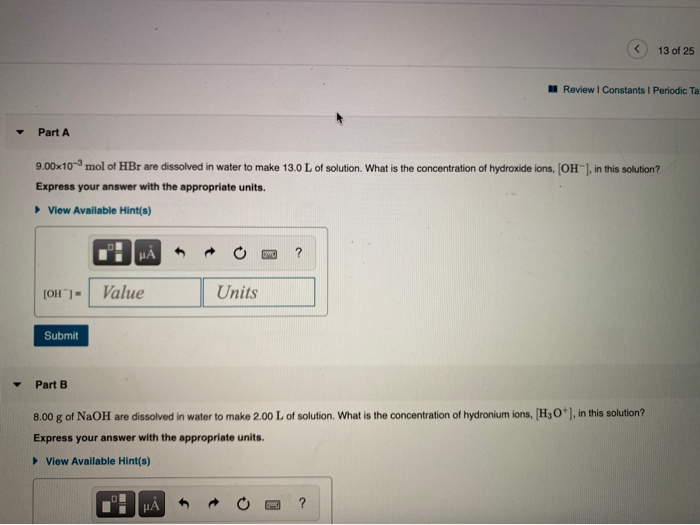13 of 25 Review | Constants Periodic Ta Part A 9.00x10- mol of HBr are dissolved in water to make 13.0 L of solution. What is the concentration of hydroxide ions, (OH), in this solution? Express your answer with the appropriate units. View Available Hint(s) MÅ [OH")- Value Units Submit Part B 8.00 g of NaOH are dissolved in water to make 2.00 L of solution. What is the concentration of hydronium ions, [H3O*), in this solution? Express your answer...